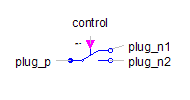Idle - MapleSim Help

Magnetic Idle

Idle running branchDescription The Magnetic Idle component is an open branch (no connection) between ${\mathrm{port}}_{p}$ and ${\mathrm{port}}_{n}$.Equations $\mathrm{\Phi }={\mathrm{\Phi }}_{p}=-{\mathrm{\phi }}_{n}=0$ ${V}_{m}={V}_{{m}_{p}}-{V}_{{m}_{n}}$Variables

 Name Value Units Description Modelica ID ${V}_{m}$ $A$ Magnetic potential difference between both ports V_m $\mathrm{\Phi }$ $\mathrm{Wb}$ Magnetic flux from port_p to port_n PhiConnections

 Name Description Modelica ID ${\mathrm{port}}_{p}$ Positive magnetic port port_p ${\mathrm{port}}_{n}$ Negative magnetic port port_nModelica Standard Library The component described in this topic is from the Modelica Standard Library. To view the original documentation, which includes author and copyright information, click here.